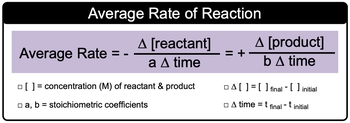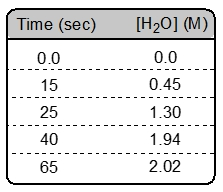## General Chemistry

Learn the toughest concepts covered in Chemistry with step-by-step video tutorials and practice problems by world-class tutors

15. Chemical Kinetics

# Average Rate of Reaction

Average (General) Rate is the change in the concentration of a compound over a period of time.

Average Rate
1
concept

## Average Rate of Reaction Concept 12m
Play a video:
now average or general rate is the change in concentration in terms of polarity overreacting a product over a period of time. Now here, when we say change in concentrations, realize that the change of concentrations for reactant will be negative in terms of their sign and for products positive. The reason for this is remember as a reaction. Proceeds reactant are lost or broken down so that we can make product. So products are made over time. With our average or general rate, we have our average or general rate expression here. We say that rate equals changing concentration over change in time. But if we're applying it to a balanced chemical equation, it takes on a little bit more meaning to it. So here we have change in concentration of reactant. Remember, change in concentration concentration is shown by brackets equals final concentration minus initial concentration divided by a times change in time. Here, A represents the studio metric coefficients for the reactant in a balanced chemical equation, this rate would equal the rate of the product formation. Here it equals positive because remember, products are being made changing product concentration divided by B times, change in time again change in product concentration is the same idea. It's final concentration minus initial concentration. Here be would represent again the story Geometric coefficient this time in terms of the products and change in time, again would be your final time, minus your initial time. So we're gonna be utilizing these general average rate expressions when looking at a balanced chemical equation and being asked to discuss the relationship in rates between your reactant and your products. Right? So keep these ideas in mind as we progress more and more into this idea of changes in concentration of are reacting molecules to produce products.2
example

## Average Rate of Reaction Example 11m
Play a video:
provide the expressions for rate of disappearance, of reactions and rate of appearance of products for the following reaction. All right, so here we're going to say that our average rate So we're gonna say our reactions are these two here. So to be change in the concentration of C three h 70 h divided by remember here, the coefficient for reactions is represented by the variable A. So for this first reactions, it's the to still be, too. Times change in time, since it's a react and it's disappearing. So to be a negative sign here That equals negative for the other. Reactant or change in 02, Divided by nine because the coefficient is nine times change in time and these are equal the products because their products are being made. So this is plus Change in concentration of co two divided by Coefficient of six. With that six times change in time equals plus for the other product being formed. Change in H20 water divided by eight times change in time. So this would represent each one of their average or general rate expressions and how they be equal to one another. So this would be your final answer
3
example

## Average Rate of Reaction Example 22m
Play a video: